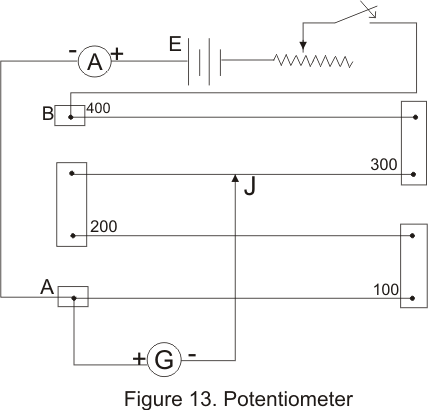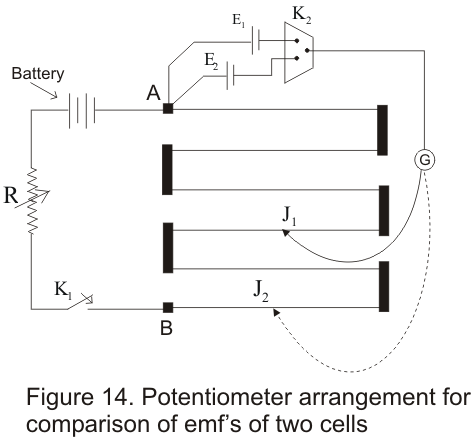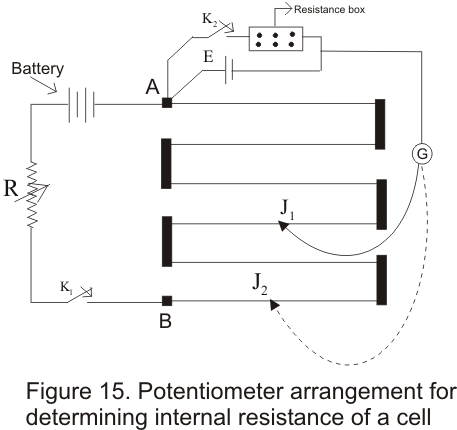# Potentiometer

Table of Content

## Potentiometer

• Potentiometer is an accurate instruments used to compare emf's of a cells,Potential difference between two points of the electric wire
• Potentiometer is based on the principle that potential drop across any portion of the wire of uniform cross-sectional is proportional to the length of that portion of the wire when a constant current flows through the wire
• Figure below shows the construction of a potentiometer which consists of a number of segments of wire of uniform area of cross-section stretched on a wooden board between two copper strips .Meter scale is fixed parallel to the lenght of the wire• A battery is connected across terminals A and B through a rheostat so that a constant currents flows through the wire
• Potentiometer is provided with a jockey J with the help of which contact can be made at any point on the wire
• Suppose A and ρ are the area of cross-section and resistivity of the material of the wire the resistance
R=ρl/A ----------------------------(i)
where l is the lenght of the wire
• If I is the current flowing through the wire then from Ohm's Law,
V=IR ------------------------------(ii)
Where V is the potential difference across the position of the wire of length l
Thus ,from (i) and (ii)
V=IR=I(ρl/A)=kl
where K=ρI/A
=> V is proportional to l when current I is constant
• K=V/l is also known as potential gradient which is the fall of potential per unit length of wire
• Sensitivity of a potentiometer depends on its potential gradient .If the potential gradient of a potentiometer is small then the potentiometer is more sensitive and hence more accurate

## (A) Comparison of EMF's of two cells using potentiometer

• Consider the circuit arrangement of potentiometer given below used for comparison of emfs of two cells• Positive terminals of two cells of emfs E1 and E2( whose emf are to be compared ) are connected to the terminals A and negative terminals are connected to jockey through a two way key K2 and a galvanometer
• Now first key K1 is closed to establish a potential difference between the terminals A and B then by closing key K2 introduce cell of EMF E1 in the circuit and null point junction J1 is determined with the help of jockey.If the null point on wire is at length
l1 from A then
E1=Kl1
Where K -> Potential gradient along the length of wire
• Similarly cell having emf E2 is introduced in the circuit and again null point J2 is determined .If length of this null point from
A is l2 then
E2=Kl2
Therefore
E1/E2=l1/l2
This simple relation allows us to find the ratio of E1/E2
• if the EMF of one cell is known then the EMF of other cell can be known easily

## (B) Determination of internal resistance of the cell

• Potentiometer can also be used to determine the internal resistance of a cell• For this a cell whose internal resistance is to be determined is connected to terminal A of the potentiometer across a resistance box through a key K2
• First close the key K1 and obtain the null point .Let l1 be the length of this null point from terminal A then
E=Kl1
• When key K2 is closed ,the cell sends current through resistance Box (R).If E2 is the terminal
Potential difference and null point is obtained at length l2(AJ2) then
V=Kl2
Thus
E/V=l1/l2
But E=I(R+ r) and V=IR
This gives
E/V=(r+R)/R
So (r+R)/R=l1/l2
giving
r=R(l1/l2-1)
• Using above equation we can find internal resistance of any given cell